# The "Plankalkül" of Konrad Zuse: A Forerunner of Today's Programming Languages

### F.L. Bauer and H. Wössner Mathematisches Institut der Technischen Universität München

The very first attempt to devise an algorithmic language...but the proposal never attained the consideration it deserved.
Heinz Rutishauser (1967)

Plankalkül was an attempt by Konrad Zuse in the 1940's to devise a notational and conceptual system for writing what today is termed a program. Although this early approach to a programming language did not lead to practical use, the plan is described here because it contains features that are standard in today's programming languages. The investigation is of historical interest; also, it may provide insights that would lead to advancements in the state of the art. Using modern programming terminology, the Plankalkül is presented to the extent it has been possible to reconstruct it from the published literature.
Key Words and Phrases: higher programming languages, programming, theory of programming, history of programming
CR Categories: 1.2, 4.22, 5.29

#### Preface

In May 1945, Konrad Zuse, Berlin-born inventor and constructor, who had arrived with his relay computer Z4 at the little village of Hinterstein in the Allgäu Alps, found himself immobilized by the postwar situation and prevented from pursuing his business. He thus found time to resume his 1943 studies,
1 on how to formulate data processing problems. Zuse understood and used the German word Rechnen, to compute, in the most general sense when he wrote, "Rechnen heisst: Aus gegebenen Angaben mach einer Vorschrift neue Angaben bilden."2
He used Angaben for data and Vorschrift for algorithm. Not having at his disposition the word Programm, he called a program Rechenplan. The notational and conceptual system for expressing a Rechenplan he called Plankalkül.
The Plankalkül, as a remarkable first beginning on the way to higher programming languages, deserves a place in the history of informatics. Although this early attempt to develop a programming language did not lead to practical use, it is nevertheless surprising to what extent the Plankalkül already contains standard features of today's programming languages.
We are led to an investigation of Zuse's Plankalkül not only because of historical interest, but also because the necessary critical reflection on the state of the art with its possible gaps and weaknesses may gain from such a study. In particular, the widespread ignorance about the Plankalkül should be diminished.
Using as a basis modern terminology in programming,3 we will describe the Plankalkül as far as it can be reconstructed from the published literature.

#### 1. Data Structure

The only primitive objects in the Plankalkül are of the mode bool (or bit), which is denoted by S0;
4 they are called Ja-Nein-Werte. Composite objects are built up recursively, in particular arrays of arbitrary dimensions and records. For example, the array modes

[0 : n - 1] bool and [0 : m - 1, 0 : n - 1] bool

are denoted by

n × S0 and m × n × S0, respectively.
If a variable indication5 (variables Strukturzeichen)or a constant indication S2 is used to denote the first of these two modes, then the second can be denoted by

m ×or m × S2, respectively.
There is also the possibility of using the abbreviated notation

S1 · n or S1 · 8

n × S0 or 8 × S0.
In this case we have a new mode bits of word length n or 8, respectively; the array, however, can still be subscripted.
A record of, say, two components, which are denoted by some variable or constant indications,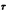or A2, A3 is specified by

(,) or (A2, A3).
Here, too, subscripts will be used for the select of components; they always start with zero.
Zuse says Strukturen for structured values and their corresponding modes; he says Art for the conglomerate consisiting of a Struktur together with its pragmatic meaning (Typ) and a possible restriction (Beschränkung), which says which of the elements of a certain structure are meaningful. For example, objects of the structure

may have the pragmatic meaning "decimal digit" and the restriction to the first 10 of the 16 lexicographic possibilities.

 A3 = ( S1 · 4 ) B3

expresses that S1 · 4 is subjected to the restriction B3.6 Zuse calls object Angaben which pretty closely corresponds to "data".
Figure 1 shows an illustrative section [Z59].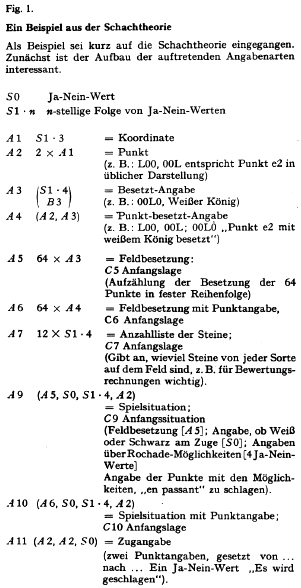#### 2. Standard Denotation

Standard denotation for Boolean obects (S0) are

L and 0

for bit sequences (for example S1 · 4)
7

LL00, L0LL.
For integers and numerical real objects, instead of bit sequences, conventional figures can also be used.8
For the standard denotation of more general, composite objects, a denotation is used which is now conventional for input and output: The standard denotations of the components of composite objects are listed in the specified order, such that the additional mode indication for the object allows one to form the decomposition uniquely. For clearness only, a special separation mark (semicolons instead of commas) is used for the seperation of composite objects.

#### 3. Free Choice of Denotation

For all objects, freely chosen identifiers (Bezeichnungen) may be introduced; for example, a standard denotation can be associated (zugeordnet) with an identifier (see
Section 6) such that both possess the same object as their value (Wert).
In a Rechenplan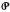, i.e. in a program or a subroutine (see Section 9), an identifier is a letter followed by a number. The letter is V, Z, R, or C, depending on whether the object in question is used as an input parameter (Variable), intermediate value (Zwischenwert), result parameter (Resultatwert), or as a constant in. The distinguishing number (Nummer) is attached to the letter in the line below. The letter classifies the objects.
Examples:

 V,0 Z,0 Z,1 R0

Finally, programs and subroutines have their own identifiers like

P12, P3·7
the number following the letter P being a program index (Programm-Index), in the form of a component-subscript (see Section 4). The second example denotes "the program 7 of the program group 3." Thus, Zuse has arrays of programs and a corresponding block structure. He derives from this a system to denote the results of subroutines in external use; for example, the result
 R0
of a subroutine P17 is external to P17 characterized by the program index 17, i.e. by
 R170
which also involves a call of P17 (see Section 8).

#### 4. Subscripting

The selection of a component is achieved with the help of a component-subscript (Komponenten-Index), that is the denotation of a number (simple subscript) or a sequence of numbers (multiple subscript). The component-subscript is written immediately under the identifying number of the corresponding composite object.
Let, for example
 V0
denote an array of the mode

l × m × S1 · n, then
 V0i (0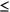i < l)
selects its ith component, a subarray of the structure m × S1 · n, while
 V0i·j (0j < m)
selects the jth component of
 V0,i
a list of the structure Sn, and finally
 V0i·j·k (0k < n)
selects the kth component of
 V0,i·j
a single bit. In today's notation, this corresponds to V0[i], V0[i, j], V0[i, j, k].

#### 5. Zuse's Two-Dimensional Notation

The form of denotation with a "main line" and "index lines" V and K for variable-number and component-subscript, respectively, is supplemented by an optional comment line S, in which the structure or mode of the value in question can be noted. To this end, the notation of
Section 1 is used; Zuse calls these indications Struktur-Indizes.
Examples are given in Figure 2, an illustrative section from [Z59, p. 69].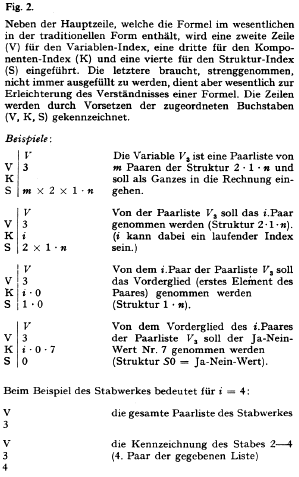The explicit marking of the lines by prefixes letters V, K, and S, allows one to omit empty K-lines. Furthermore, the prefix S in the mode denotation can be droppped. Thus,
S| Sn m × Sn S0 S2can be shortened to
S| 1·n m × 1·n 0 2Moreover, Zuse allows the abbreviation of
S| A1 A2 S0 A3by
A| 1 2 0 3(using A0 synonymously with S0)

Furthermore, variable component subscripts can be used [Z70, p. 123], for example by the help of an intermediate value in the form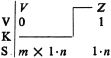with the meaning of V0[Z1] in today's notation. (Note that Z1 is of structure Sn; that is, the integer corresponding to the bit sequence Z1 is used as subscript, and a component of structure Sn is selected from V0.)

#### 6. Assignment and Identity Declarations

The most important feature for the construction of programs is the assignement (Rechenplangleichung), expressed by means of the Ergibizeichen =>
9 For example, the assignment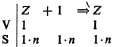means to augment the integer intermediate value Z1 by 1, while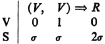means the composition of the values V0 and V1 to a composite value, which is denoted by R0.
The interpretation of the second example shows that the assignment comprises the semantic meaning of an (initialized) identity declaration for a variable: The identifier R0 of the mode 2is used to denote the elaborated value on the left-hand side.
If in a program more than one assignment to the same result or intermediate value variabke occurs, then the (dynamically) first assignment is to be interpreted as an (initialized) identity declaration for a variable, while all others are ordinary assignments. This would give the genuine concept of a variable. On the other hand, the initialization of an input parameter in connection with a subroutine call,10 as well as the intitialization of constants, can be interpreted to be an ordinary identity declaration. However, these fine distinctions are reflected neither in the notation nor in the explanation of the semantics [Z59, p. 70]. Nevertheless, they have strongly influenced Rutishauser's ideas, as seen from ALGOL 58.
The usual arithmetic and Boolean operations are provided for, and they allow one to form expressions (Ausdrücke) in connective formula notation.11 Besides, comparison operations like =,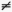,, with Boolean values as results, can be used. For arithmetic operations, objects of the mode bits (denoted by Sn) are interpreted as numbers in direct (lexicographic) coding.

[? Der Operator jux hat grosse Vortelle bet der systematischen Untersuchung einer sich evrl. In threm Umfang kiufend ünderzden Liste auf Glieder einer bestimmien Eigenschaft und Verarbdung derseiben. ?]

#### 7. Further Operational Features

Apart from the possibility of selecting record and array components by (component) subscripts, certain operations from the predicate calculus are used to test components with respect to a specified property, with the result of selecting them or of obtaining a Boolean value. In this respect the Plankalkül surpasses the potentialities in today's programming languages, including ALGOL 68.
Zuse uses both the "existence" and the "all" operator, and in particular the operator µ:
 µx(xV ^ R(x)) 0
means "The next component of V0, for which the property R holds."
The property R, in the notation R(x), is expressed by means of a computational rule which gives a Boolean values (Ja-Nein-Wert), or of a result parameter of a suitable subroutine (see
Section 8).
It is clear, that procedures can be defined in, say, ALGOL 68, which have the above effect. But it may be worthwhile to see whether Zuse's constructions could be introduced as original concepts in high level languages. See also [BG72].

#### 8. Statements and Subroutine Calls

Statements are what Zuse calls Planteile. In particular, assignments are statements. Other statements, which we shall discuss, are conditional statements and repetitive statements. There is also a compound statement, formed with the help of parenthesis. In order to seperate statements, as well as the line marks (see
Section 5), a vertical bar is used.
Conditional statements are formed with the help of the Bedingt-Zeichen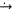(or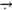) in the following form
ßA
where the condition (Bedingung) ß is an expression with Boolean value, and A an arbitrary statement. The elaboration of this conditional statement (bedingter Planteil) begins with ß and ends with ß or is continued with A, depending on whether ß produces the value 0 = nein or L = ja. An alternative for A in the first case cannot be specified.
The following example of a repetitive statement, that is initiated by the letter W, shows an application of the µ-operation of the preceding section:The elaboration of this Wiederholungsplan starts with the first assignment. The left-hand side formula of this assignment produces at each elaboration the next component V0[i] which is different from V1, provided it exists. In this case the following statement is elaborated and the process starts again. If, however, no component is found then Z0 is unchanged and the elaboration of the repetitive statement is finished.
In the second assignment of this example, where an initialization of R0 is presupposed, R171(Z0) is the call of a subroutine P17 (see Section 9), which is specified to have one input parameter and a result parameter R1 (see Section 3). The elaboration of this call means the identification of the actual parameter Z0 with the formal input parameter, and following this, the elabotation of P17. The value of the call is the value which is obtained by R1.
If it was initialized by L, R0 obtains thus, when the repetitive statement is finished, the value of the conjunction of all R171(x) where x is from the set of all elements of V0 that are different from V1.

#### 9. Programs

Both programs and subroutines in the Plankalkül are expressed in the form of procedures (Rechenpläne); i.e. they are prefaces by a specification part (Randauszug), which specifies the parameters as being input parameters or result parameters together with their modes. The computatational rules proper is then described in the body (Anweisungsteil), which consists of a sequence of statements. The end is marked by a symbol FIN.
12
A call requires that the actual parameters have consistent mode. The subroutine P17 that was called in the preceding section may begin with the following specification part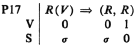where V0 is an input and R0, R1 are result parameters. The body must contain assignments to R0 and R1. If it contains intermediate values, then they are not readable directly outside of P17.

[ ? Mein 'Plankalkül' war doch inzwischen lüngst veraltes. ? ]

-- K. Zuse (1970)

#### 10. ALGOL 68 Translation of Some Plankalkül Programs

It should not be forgotten that Zuse did not only invent the Plankalkül, but that he used it to formulate some nontrivial programs of the nonnumerical kind (he called them logistisch-kombinativ) in order to demonstrate the potentialities of computing. The programs are by all means nontrivial for the year 1945 and more ambitious than the first task steps von Neumann did with his Gedanken machine (cf. [K70]). To illuminate this, we give in the following ALGOL 68 transcriptions of program examples from [Z49] and [Z59].

#### a. Syntax Checking for Boolean Expressions

A typical application of the Plankalkül [Z49, p. 446] contains a procedure for the syntax check of Boolean expressions. Zuse starts from the observation:
Such expressions contain the following symbols: variable symbols, negation symbol, operation symbols, parentheses symbols, and space symbol that is needed for the separation of expressions. The symbols in question are coded in bit sequences.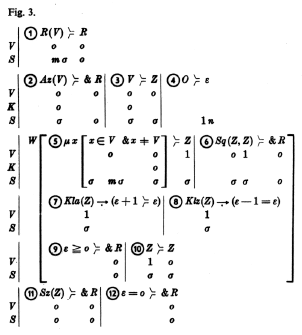In the procedure (Figure 3),denotes the structure of these 8-bit sequences, and mwith arbitrary m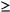1 denotes the symbol sequences that are to be investigated. A call of the procedure with a (coded) symbol sequence x as its actual parameter means to test the predicate
Sa(x): <<x is a 'meaningful expression', i.e. a (syntactically correct) Boolean expression>>.
This predicate is introduced recursively by:
(i) A variable symbol is a meaningful expression.
(ii) A meaningful expression, prefixed by a negation symbol, yields a meaningful expression.
(iii) Two meaningful expressions, connected by an operation symbol, yield a meaningful expression.
(iv) A meaningful expression, put in parentheses, yields a meaningful expression.
To transform this definition into an algorithm, Zuse defines, now for symbols x, the auxilliary predicates:
Va(x): <<x is a variable symbol>>
Op(x): <<x is operation symbol>>
Neg(x): <<x is negation symbol>>
Kla(x): <<x is opening patenthesis>>
Klz(x): <<x is closing parenthesis>>
and furthermore the predicates:
Az(x): Va(x) V Neg(x) V Kla(x)
Sz(x): Va(x) V Klz(x)
Sq(x,y): (Sz(x) ^ ¬ Az(y)) V (¬Sz(x) ^ Az(y))
He then postulates:
1. The first symbol x has to fulfill Az(x)
2. Two symbols x, y following each other have to fulfill Sq(x, y)
3. The last symbol x has to fulfill Sz(x).
Moreover, he uses the two parentheses counts:
4. The number of opening parenthesis has to be equal to the number of closing parentheses.
5. For any segment of the symbol sequences, the number of opening parentheses must not be smaller than the number of closing parentheses.

The program (Figure 3) checks these conditions: (2) serves for the special case of condition 1. (3) and (4) are initializations for the repetitive statement which checks condition 2 and the count 5. Condition 3 for the final case is then checked in (11) and the count 4 in (12). The program, by the way, contains mistakes: for example, a count corresponding to (7) is missing for the first symbol. More seriously, the condition xV0 in (5) should be read as x = V0[i] ^ i0.

For a direct transliteration of Zuse's (corrected) procedure, we assume first that suitable Boolean procedures Va(x), Op(x), etc., are declared. Using these predicates, we obtain in ALGOL 68 (the encircled numbers refer to Figure 3):

(1) proc Sa = ([0 : either] bits V0) bool : begin
(2) bits Z0 := V0;
(3) bool R := Az(Z0);
(4) int eps := 0; if Kla(Z0) then eps := 1 fi;
(5) for i to upb V0 while R do begin
bits Z1 := V0[i];
(6) R := R ^ Sq(Z0, Z1);
(7) if Kla(Z1) then eps +:= 1 fi; (8) if Klz(Z1) then eps -:= 1 fi; (9) R := R ^ eps >= 0;
(10) Z0 := Z1 end;
(11), (12) R ^ Sz(Z0) ^ eps = 0 end
(Of course, in ALGOL 68 there exist possibilities for a more efficient formulation.)

#### b. Checking a Move of the White KingFigure 4 shows one of the auxiliary procedures for a chess program formulated by Zuse in Plankalkül notations [Z59, p. 71]. The modes that are found in the program are seen from Figure 1 (note that A5 and A6 are to be interchanged). Zuse's procedure, directly transliterated into ALGOL 68 (the numbers 1 to 4 correspond to those in Figure 4) reads as follows:
 mode A1 = int co coordinates 1, ..., 8 instead of 0, ..., 7 corresponding to [0:2] bool co, \ A2 = [1:2] A1 co point co, A3 = int co occupation by 1, ..., 6 (9, ..., 14) for white (black) Q, K, R, B, S, P; instead of 0 for unoccupied co, A4 = struct (A2 point, A3 occ) co occupation of the point co, A5 = [1:64] A4 co occupation of the board co; proc R17 co adjacent co = (A2 V0, V1) bool : abs (V0 - V1) <= 1 ^ abs (V0 - V1) <= 1; proc R128 co move permissible co = (A5 V0, A2 V1, V2) bool : <> ^ <>; 1) proc R148 co move 2 (wK) permissible co = (A5 V0, ref A2 px) bool : co additional result parameter px for reference to target co begin bool c co if already checked, px refers to permissible target co := false; 2) int i := 1; while occ of V0[i]2 do i +:= 1; A4 Z0 = V0[i]; 3) for j to 64 while ¬c do    begin A4 x = V0[i]; px := point of x;       c := R17 (point of Z0, px) ^ occ of x >= 8; 4) for k to 64 while c do       begin A4 y = V0[k];          if occ of y > 8 then c := ¬R128 (V0, point of y, px) fi       end    end;    c end

[ ? Troszdern glaube ichm dass der... Plankalkül noch einmel praktische Bedeutung bekommen wird. ? ]

#### Concluding Remarks

Altogether the Plankalkül turns out to be a highly developed programming languag with structured objects that are built from a single primitive mode of objects - the two Boolean values (Ja-Nein-Werte) 0, L. Conceptually, this is certainly advantageous, but the existing plurality of modes in some predominant programming languages indicates the practical weakness of this approach. Apart from this, the Plankalkül show many of the features of the programming languages of the sixties, sometimes obscured by an unorthodox notation, which disregarded some requirements of mechanical processing as well as some of the common notational habits. Some features - for example the structuring of objects - have only recently come into existing programming languages; others have yet to come. In particular, consideration of the features mentioned in Section 7 could be rewarding.
To assess the Plankalkül historically, one has to compare it with the flow diagram symbolism that originated at about the same time in the United States. Zuse's pioneering achievement of the forties should not be diminished by certain limitations, e.g. that the specification of modes is meant only to be an informal help for the correct use (in particular with respect to the parameters) of a procedure and not an intrinsic part of the program, or that the explicit formation of all modes from a single basic mode as well as the corresponding notation, are clumsy, or that questions of implementation have not been tackled13.
It is also interesting to indicate the features that are generally accepted today but which were not contained in the Plankalkül. Here we should first mention the reference concept - it is not even obvious whether => means an identity declaration or an assignment. Names or references as objects are also missing in ALGOL 60; in this respect the relation between Plankalkül and Rutishauser's influence14 on ALGOL 60 is obvious. The essential restriction to numerical objects in ALGOL 60 was, as one knows today, not critical; the intention was to make the address calculation not accessible to the programmer, and this was motivated by the desire for error-free programming as well as by awareness of the frequent malfunction of machines in those years.15 Thus, at that time, there was not enough justification to open, in ALGOL 60, the Pandora's box of manipulable names - i.e. addresses.16 It was therefore left to Wirth to introduce this later into higher programming languages, and it can now be found in ALGOL 68 as well as in some "lower level languages."

#### References

Z43.
Zuse, K. Ansätze einer allgemeinen Theorie des Rechnens. 1943, unpublished.
Z45.
Zuse, K. "Plankalkül", Theorie der angewandten Logistik. 1945, unpublished.
Z49.
Zuse, K. Über den allgemeinen Plankalkül als Mittel zur Formulierung schematisch-kombinativer Aufgaben. Archiv Math. I (1948/49), 441-449. (Received Dec 6, 1948.)
Z49a.
Zuse, K. Die mathematischen Voraussetzungen für die Entwicklung logistisch-kombinativer Rechenmaschinen. ZAMM 29 (1949), 36-37. (Lecture, GAMM Conference Göttingen, Sept. 1948.)
R52.
Rutishauser, H. Automatische Rechenplanfertigung bel programmgestewerten Rechenmuschinen. Mitteilungen aus dem Institut für angewandte Mathematik der ETH Zürich, No. 3. Birkhäuser, Basel, 1952.
Z59.
Zuse, K. Über den Plankalkül, Elektron. Rechenanl. I (1959), 68-71.
Z68.
Zuse, K. Gesichtspunkte zur sprachlichen Formulierung in Vielfachzugriffssystemen unter Berücksichtigung des Rechensysteme. Oldenbourg, Munich 1968.
Z70.
Zuse, K. Der computer mein Lebenswerk. Verlag Moderne Industrie, Munich, 1970.
K70.
Knuth, D. E. Von Neumann's first computer program. Computing Surveys 2 (1970), 247-260.
BG71.
Bauer, F. L., and Goos, G. Informatik: Eine einführende Ubersicht. Springer, Berlin, 1971.
BG72.
Bauer, F. L., and Gnatz, R.

1 "Anzätze einer Theorie des allgemeinen Rechnens," a planned Ph.D. dissertation. See [Z70, p. 112].

2 See [Z49].

3 In terminology and notation, we follow ALGOL 68. Whatever position one may have with respect to ALGOL 68, the difference from other reputable terminologies and notations, such as the one Hoare, Wirth and Dijkstra prefer, is not so great that it would hinder communication.

4 In [Z49] a small o is used.

6 For a chess example such a restriction is defined in [Z59, p. 72] by: "A3 is restricted to 13 possibilities: 12 kinds of chessmen and o for unoccupied."

7 [Z59, p. 70]. From a remark in [Z70, p. 157], one can infer that Zuse already during his Berlin period, that is before 1944, used L and 0, which he called Sekundalziffern (see also [Z70, p. 68] in his diary entry of June 20, 1937).

8 It should be noted that Zuse already used floating point computation.

9 Originally, Zuse [Z59] introduced the >==, shaped equality sign. The arrow-like sign => is used in [Z59], after Rutishauser had helped to propagate it. In [R52], Rutishauser used in typescript the sign =>=. At the Zürich ALGOL Conference 1958, the sign := was introduced under strong pressure from the American participants. The European group wished to use Zuse's sign.

10 It cannot be excluded that Zuse considered the input parameters to be genuine variables whose values can be changed during the subroutine. This is indicated by an isolated occurance of (V/5, V/6) => (V/7) in [Z59].

11 [Z49, p. 447]: "The Ergibt-Zeichen >== joins an expression which is to be calculated (left) with a result (right)." According to Zuse such expressions mean computational rules (Rechenvorschritten.)

12 The example in [Z59, p. 71] ends, however, with an expression E instead of E ==> Ro FIN, where Ro is the only result parameter.

13 K. Zuse in [Z70, p. 128]: "Der Plankalkül hätte noch 'compiler-gerecht' zugeschnitten werden müssen."

14 F.L. Bauer. Heinz Rutishauser, Nachruf. Computing 7 (1971), 129-130.

15 "By this token one can calculate addresses. Symbolically, one can bring about this feature by a single wire. I had misgivings to do this step." [Z70, p. 99.]

16 The question was, by the way, violently discussed at the Paris ALGOL Conference in January 1960. Proponent of "generated names" was Julian Green, who wanted ALGOL to have the possibility of describing it's own translator.

Copyright (c)1972, Association for Computing Machinery, Inc.
General permission to republish, but not for profit, all or part of this material is granted, provided that reference is made to this publication, to it's date of issue, and to the fact that reprinting privileges were granted by permission of the Association for Computing Machinery.
Author's address: Mathematisches Institut der Technischen Universität München, 8000 München 2, Postfach 202420, Germany.

Republished from Communications of the ACM, July 1972, Volume 15, Number 7. Reprinting privileges were granted by permission of the Association of Computing Machinery.
Last updated Oct 1998,
Cat's-Eye Technologies. Any inaccuracies from the original document are our fault and we would appreciate being notified of any errors.